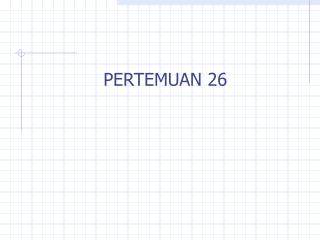# PERTEMUAN 26 - PowerPoint PPT PresentationDownload PresentationPERTEMUAN 26

PERTEMUAN 26Download Presentation## PERTEMUAN 26

- - - - - - - - - - - - - - - - - - - - - - - - - - - E N D - - - - - - - - - - - - - - - - - - - - - - - - - - -
##### Presentation Transcript

1. PERTEMUAN 26

2. Markov Chains and Random Walks

3. Fundamental Theorem of Markov Chains If Mg is an irreducible, aperiodic Markov Chain: • All states are positive reccurent. • Pk converges to W, where each row of W is the same (and equal to , say) •  is the unique vector for which P = 

4. Fundamental Theorem of Markov Chains • Let Mg be a Markov Chain with states S0…Sn • The Fundamental Theoremtells us that after a sufficiently large number of time steps, the probability of being in state Si+1 is the same as being in state Si. • This steady-state condition is known as a stationary distribution • The rate at which a Markov Chain converges to a stationary distribution is called the mixing rate.

5. Random Walks • A Random Walk on connected, undirected, non-bipartite Graph G can be modeled as a Markov Chain Mg, where the vertices of the Graph, V(G), are represented by the states of the the Markov Chain and the transition matrix is as follows If (u,v) is a member of E otherwise

6. Random Walks • Mg is irreducible because G is connected • Mg is aperiodic • Periodicity is the GCD of the length of all closed walks on G • Since G is undirected, there exist closed walks of length 2 (u,v  E, exists walk u-v-u) • Since G is non-bipartite it contains odd cycles • Therefore GCD of all closed walks is 1 • Mg is aperiodic

7. Random Walks • Given that Mg is aperiodic and irreducible, we can apply the Fundamental Theorem of Markov Chains and deduce that Mg converges to a stationary distribution. • Lemma: • For all v  V, v = d(v) / 2 |E| ( d(v) = the degree of v) • Proof denote the component corresponding to vertex v in the probability vector

8. Hitting time (huv) – expected number of steps in a Random Walk that starts at u and ends upon its first visit to v • Commute time (cuv) -- expected number of steps in a Random Walk that starts at u, visitsv once and returns to u. (cuv = huv + hvu)

9. The Lollipop Graph • Lollipop Graph consists of n vertices • A clique on n/2 vertices • A path on n/2 vertices • Let u,v  V, u is in the clique, v is at the far end of the path. • Surprisingly, huv != hvu (huv is (n3) hvu is  (n2)

10. Markov Chains: an Application • Link Prediction and Path Analysis using Markov Chains • Use Markov Chains to perform probabilistic analysis and modeling of weblink sequences; ie. If a user requests page n, what will be her most likely next choice • Possible Applications • Web Server Request Prediction • Adaptive Web Navigation • Tour Generation • Personalized Hub • Model can be used in adaptive mode; transition matrix can be updated as new data (example: Web Server Request) arrives

11. Markov Chains: an Application Link Prediction and Path Analysis using Markov Chains System Overview

12. Markov Chains: an Application Experimental Results HTTP Server Request Prediction • 6572 URIs (including html documents, directories, gifs, and cgi requests) • 40,000 Requests • Over 50% of the web server requests can be predicted to be the state with the highest probability

13. References • L. Lovasz. Random Walks on Graphs: A Survey. Combinatorics: Paul Erdos is Eighty (vol. 2), 1996, pp. 353-398. (http://www.cs.yale.edu/HTML/YALE/CS/HyPlans/lovasz/erdos.ps) • R. Sarukkai, "Link Prediction and Path Analysis Using Markov Chains: 9th World Wide Wide Conference, May, 2000. (http://www9.org/w9cdrom/68/68.html) • Introduction to Markov chains : with special emphasis on rapid mixing / Ehrhard Behrends. Germany [1990-onward] Vieweg & Sohn, GW Am Math Soc 2000.